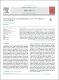## Measurement of B-s(0) meson production in pp and PbPb collisions at root S-NN=5.02 TeV2019
Elsevier
##### Abstract

The production cross sections of ({\text{B}}{\text{s}}^{0}) mesons and charge conjugates are measured in proton-proton (pp) and PbPb collisions via the exclusive decay channel ({\text{B}}{\text{s}}^{0}\to \text{J}/\psi \varphi \to {\mu }^{+}{\mu }^{-}{\text{K}}^{+}{\text{K}}^{-}) at a center-of-mass energy of 5.02 TeV per nucleon pair and within the rapidity range (|y|<2.4) using the CMS detector at the LHC. The pp measurement is performed as a function of transverse momentum (({p}{\text{T}})) of the ({\text{B}}{\text{s}}^{0}) mesons in the range of 7 to 50 GeV/c and is compared to the predictions of perturbative QCD calculations. The ({\text{B}}{\text{s}}^{0}) production yield in PbPb collisions is measured in two ({p}{\text{T}}) intervals, 7 to 15 and 15 to 50 GeV/c, and compared to the yield in pp collisions in the same kinematic region. The nuclear modification factor (({R}{\text{AA}})) is found to be (1.5±0.6\left(\text{stat}\right)±0.5\left(\text{syst}\right)) for 7–15 GeV/c, and (0.87±0.30\left(\text{stat}\right)±0.17\left(\text{syst}\right)) for 15–50 GeV/c, respectively. Within current uncertainties, the ({\text{B}}{\text{s}}^{0}) results are consistent with models of strangeness enhancement, and suppression by parton energy loss, as observed for the ({\text{B}}^{+}) mesons.

##### Keywords
Physics, Quark gluon plasma, B meson, Open heavy-flavor, ENERGY, DEPENDENCE, KAON, Astronomy & Astrophysics, Physics, Nuclear, Physics, Particles & Fields
##### Citation
Sirunyan, A. M., Tumasyan, A., Adam, W., Ambrogi, F., Asilar, E., Bergauer, T., Brandstetter, J., Dragicevic, M., Erö, J., Escalante Del Valle, A., Flechl, M., Frühwirth, R., Ghete, V. M., Hrubec, J., Jeitler, M., Krammer, N., Krätschmer, I., Liko, D., Madlener, T., … Woods, N. (2019). Measurement of Bs0 meson production in pp and PbPb collisions at \sqrt{s_{\mathrm{NN}}}= 5.02 TeV. In Physics Letters B (Vol. 796, pp. 168–190). Elsevier BV. https://doi.org/10.1016/j.physletb.2019.07.014